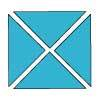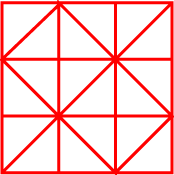#### You may also like### Chocolate

There are three tables in a room with blocks of chocolate on each. Where would be the best place for each child in the class to sit if they came in one at a time?### Four Triangles Puzzle

Cut four triangles from a square as shown in the picture. How many different shapes can you make by fitting the four triangles back together?### Cut it Out

Can you dissect an equilateral triangle into 6 smaller ones? What number of smaller equilateral triangles is it NOT possible to dissect a larger equilateral triangle into?

# Fractional Triangles

##### Age 7 to 11 Challenge Level:

Use the lines on this figure to show how the pattern of triangles can be used to divide the square into two halves, three thirds, six sixths and nine ninths.More lines are needed to divide it into four quarters.

What is the least amount of line needed to do this if the quarters are in one piece and all the same shape?

How many ways can you divide it into halves using just the lines given?

You might like to try out your ideas below.
Click on a dot to choose a colour, then click on a triangle.